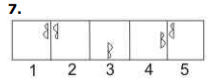# BITSAT Logical Reasoning Test - 2

## 10 Questions MCQ Test BITSAT Subject Wise & Full Length Mock Tests | BITSAT Logical Reasoning Test - 2

Description
This mock test of BITSAT Logical Reasoning Test - 2 for JEE helps you for every JEE entrance exam. This contains 10 Multiple Choice Questions for JEE BITSAT Logical Reasoning Test - 2 (mcq) to study with solutions a complete question bank. The solved questions answers in this BITSAT Logical Reasoning Test - 2 quiz give you a good mix of easy questions and tough questions. JEE students definitely take this BITSAT Logical Reasoning Test - 2 exercise for a better result in the exam. You can find other BITSAT Logical Reasoning Test - 2 extra questions, long questions & short questions for JEE on EduRev as well by searching above.
QUESTION: 1

### Complete the analogous pair. Court : Justice : : School : ?

Solution:

First is the place where the second is imparted.

QUESTION: 2

### Choose the word which is least like the other words in the group .

Solution:

Apple, Mango and orange are fruits. But potato is a vegetable .

QUESTION: 3

### Group the following figures into three classes on the basis of identical properties.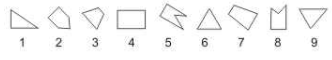Solution:

1,6,9, are all triangles.
3,4,7, are all four-sided figures.
2,5,8 are all five-sided figures.

QUESTION: 4

Find the missing character.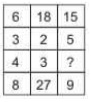Solution: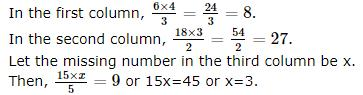QUESTION: 5

In this letter series, some of the letters are missing. Choose the correct letter given below -
_ baa _ aab _ a _ a

Solution:

_ baa _ aab _ a _a
A) a baa a aab b a b a
B) a baa a aab b a a a
C) a baa b aab a a b a
D) b baa a aab a a b a
Here, only Option C looks like a pattern.

QUESTION: 6

Find out the term in the number series which is wrong.
0, 3, 8, 15, 24, 33

Solution:

The difference between the sequence 0, 3, 8, 15, 24, 33 is  3, + 5, + 7, + 9, + 11.

So 33 is wrong.

QUESTION: 7

Each of the following questions consists of five Problem figures marked 1,2,3,4 and 5 followed by five Answer figures marked A, B, C, D, and E. Select a figure from the Answer Figures which will continue the same series as given in the Problem figures.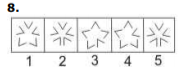Solution:
QUESTION: 8

Select the correct mirror-image of the Figure (X) from amongst the given alternatives.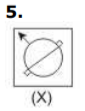Solution:
QUESTION: 9

Select the correct mirror-image of the Figure (X) from amongst the given alternatives.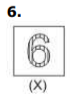Solution:
QUESTION: 10